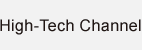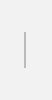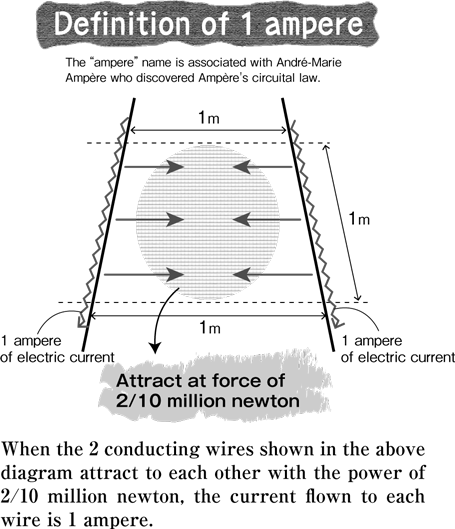### One of the most basic units about electricity

Symbol: A / Base data: electric current. SI base units.
Universal electric current is when a current is flown through two infinitely long linear conductors with infinitely small circular cross-sectional areas placed in parallel at intervals of 1 meter in a vacuum state, and these conductors exert force of 2×10-7 Newton on each other every 1 meter.

Definition based on force mutually exerted by two conductors

When an electric current is flown in parallel through two electrical wires, this generates forces that are mutually attracted and repulsed. The stronger the current the stronger the force, and vice versa. This action is used to define “1 ampere” of electric current.